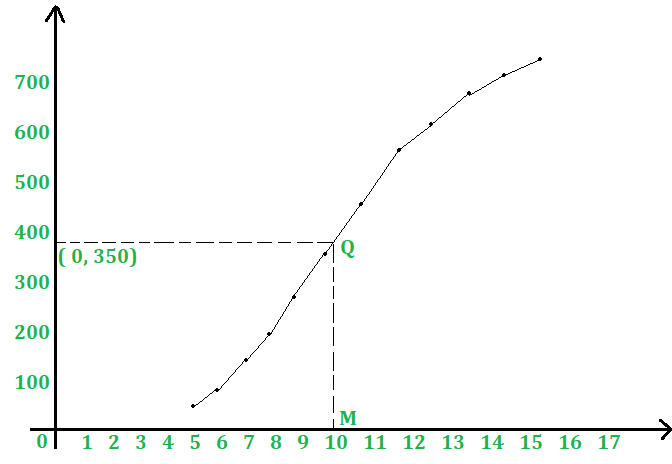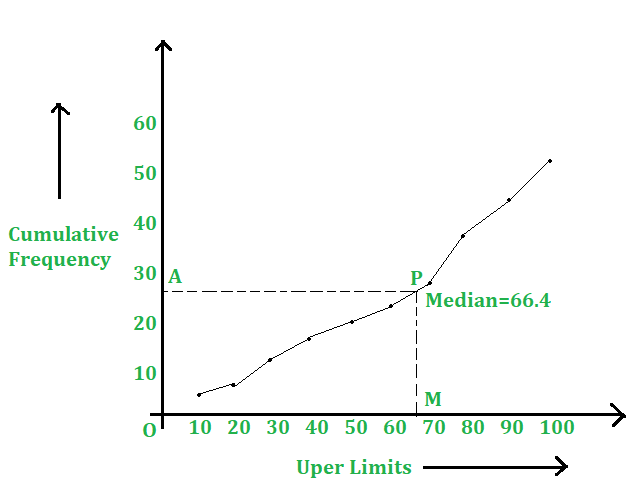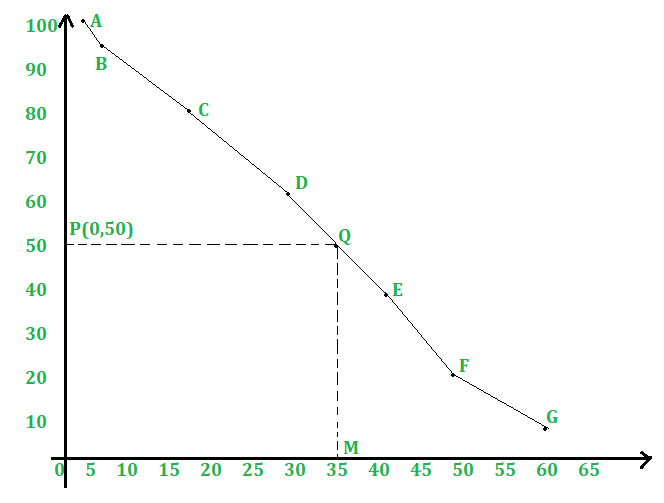Open in App
Not now

# Mean, Median and Mode of Grouped Data

• Difficulty Level : Medium
• Last Updated : 02 Nov, 2022

Suppose we want to compare the age of students in two schools and determine which school has more aged students. If we compare on the basis of individual students, we cannot conclude anything. However, if for the given data, we get a representative value that signifies the characteristics of the data, the comparison becomes easy.

A certain value representative of the whole data and signifying its characteristics is called an average of the data. Three types of averages are useful for analyzing data. They are:

• Mean
• Median
• Mode

In this article, we will study three types of averages for the analysis of the data.

## Mean

The mean (or average) of observations is the sum of the values of all the observations divided by the total number of observations. The mean of the data is given by x = f1x1 + f2x2 + …. + fnxn/f1 + f2+… + fn. The mean Formula is given by,

Mean = ∑(fi.xi)/∑fi

## Methods for Calculating Mean

### Method 1: Direct Method for Calculating Mean

Step 1: For each class, find the class mark xi, as

x=1/2(lower limit + upper limit)

Step 2: Calculate fi.xi for each i.

Step 3: Use the formula Mean = ∑(fi.xi)/∑fi.

Example: Find the mean of the following data.

Solution:

We may prepare the table given below:

Mean = ∑(fi.xi)/∑fi = 1100/50 = 22

### Method 2: Assumed – Mean Method For Calculating Mean

For calculating the mean in such cases we proceed as under.

Step 1: For each class interval, calculate the class mark x by using the formula: xi = 1/2 (lower limit + upper limit).

Step 2: Choose a suitable value of mean and denote it by A. x in the middle as the assumed mean and denote it by A.

Step 3: Calculate the deviations di = (x, -A) for each i.

Step 4: Calculate the product (fi x di) for each i.

Step 5: Find n = ∑fi

Step 6: Calculate the mean, x, by using the formula: X = A + ∑fidi/n.

Example: Using the assumed-mean method, find the mean of the following data:

Solution:

Let A=25 be the assumed mean. Then we have,

Mean = X = A + ∑fidi/n = (25 + 110/50) = 27.2

### Method 3: Step-Deviation method for Calculating Mean

When the values of x, and f are large, the calculation of the mean by the above methods becomes tedious. In such cases, we use the step-deviation method, given below.

Step 1: For each class interval, calculate the class mark x, where X = 1/2 (lower limit + upper limit).

Step 2: Choose a suitable value of x, in the middle of the x, column as the assumed mean and denote it by A.

Step 3: Calculate h = [(upper limit) – (lower limit)], which is the same for all the classes.

Step 4: Calculate ui = (xi -A) /h for each class.

Step 5: Calculate fu for each class and hence find ∑(fi × ui).

Step 6: Calculate the mean by using the formula: x = A + {h × ∑(fi × ui)/ ∑fi}

Example: Find the mean of the following frequency distribution:

Solution:

We prepare the given table below,

A = 100, h = 20, ∑fi = 100 and ∑(fi x ui) = 61

x = A + {h × ∑(fi x ui) / ∑fi}

=100 + {20 × 61/100} = (100 + 12.2) =112.2

## Median

We first arrange the given data values of the observations in ascending order. Then, if n is odd, the median is the (n+1/2). And if n is even, then the median will be the average of the n/2th and the (n/2 +1)th observation. The formula for Calculating Median:

Median, Me = l + {h x (N/2 – cf )/f}

Where,

• l = lower limit of median class.
• h = width of median class.
• f = frequency of median class,
• cf = cumulative frequency of the class preceding the median class.
• N = ∑fi

Example: Calculate the median for the following frequency distribution.

Solution:

We may prepare cumulative frequency table as given below,

Now, N = 80 = (N/2) = 40.

The cumulative frequency just greater than 40 is 58 and the corresponding class is 24-32.

Thus, the median class is 24-32.

l = 24, h = 8, f = 24, cf = c.f. of preceding class = 34, and (N/2) = 40.

Median, Me = l+ h{(N/2-cf)/f}

= 24 + 8 {(40 – 34)/ 24}

= 26

Hence, median = 26.

## Mode

It is that value of a variety that occurs most often. More precisely, the mode is the value of the variable at which the concentration of the data is maximum.

Modal Class: In a frequency distribution, the class having the maximum frequency is called the modal class. The formula for Calculating Mode:

Mo = xk + h{(fk – fk-1)/(2fk – fk-1 – fk+1)}

Where,

• xk = lower limit of the modal class interval.
• fk = frequency of the modal class.
• fk-1= frequency of the class preceding the modal class.
• fk+1 = frequency of the class succeeding the modal class.
• h = width of the class interval.

Example 1: Calculate the mode for the following frequency distribution.

Solution:

Class 40-50 has the maximum frequency, so it is called the modal class.

xk = 40, h = 10, fk = 28, fk-1 = 12, fk+1 = 20

Mode, Mo= xk + h{(fk – fk-1)/(2fk – fk-1 – fk+1)}

= 40 + 10{(28 – 12)/(2 × 28 – 12 – 20)}

= 46.67

Hence, mode = 46.67

Important Result

Relationship among mean, median and mode,

Mode = 3(Median) – 2(Mean)

Example 2: Find the mean, mode, and median for the following data,

Solution:

We have,

Mean = ∑(fi.xi)/∑f

= 2640/100

= 26.4

Here, N = 100 ⇒ N / 2 = 50.

Cumulative frequency just greater than 50 is 60 and corresponding class is 20-30.

Thus, the median class is 20-30.

Hence, l = 20, h = 10, f = 36, c =  c. f. of preceding class = 24 and N/2=50

Median, Me = l + h{(N/2 – cf)/f}

= 20+10{(50-24)/36}

Median = 27.2.

Mode = 3(median) – 2(mean) = (3 × 27.2 – 2 × 26.4) = 28.8.

## Ogives

Let a grouped frequency distribution be given to us. On graph paper, we mark the upper-class limits along the x-axis and the corresponding cumulative frequencies along the y-axis. By joining these points successively by line segments, we get a polygon, called a cumulative frequency polygon. On joining these points successively by smooth curves, we get a curve, known as ogive.

The step-by-step process for the construction of an Ogive graph:

1. First, we mark the upper limits of class intervals on the horizontal x-axis and their corresponding cumulative frequencies on the y-axis.
2. Now plot all the corresponding points of the ordered pair given by (upper limit, cumulative frequency).
3. Join all the points with a free hand.
4. The curve we get is known as ogive.### Types of Ogives

• Less Than Ogive

On graph paper, we mark the upper-class limits along the x-axis and the corresponding cumulative frequencies along the y-axis.

1. On joining these points successively by line segments, we get a polygon, called a cumulative frequency polygon.
2. On joining these points successively by smooth curves, we get a curve, known as less than ogive.• More Than Ogive

On graph paper, we mark the lower class limits along the x-axis and the corresponding cumulative frequencies along the y-axis.

1. On joining these points successively by line segments, we get a polygon, called the cumulative frequency polygon.
2. On joining these points successively by smooth curves, we get a curve, known as more than ogive.My Personal Notes arrow_drop_up